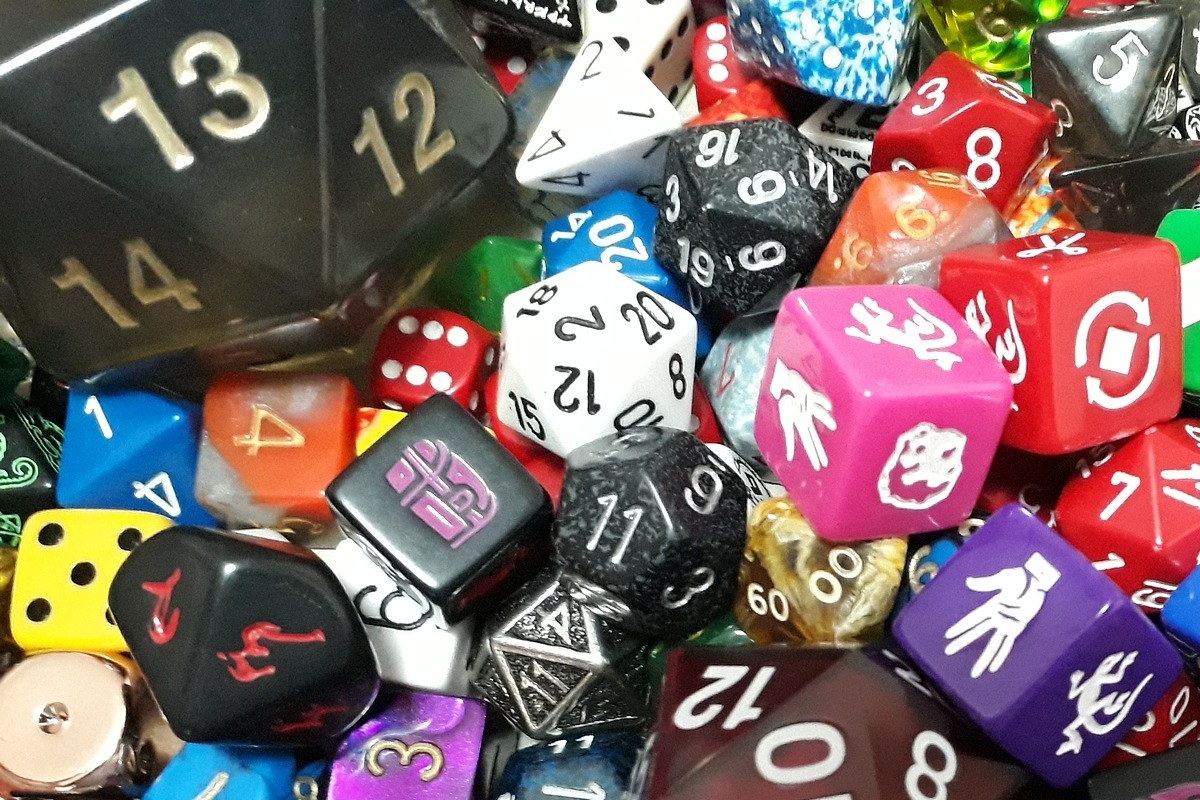# What are Math Dice and How Can You Use Them in Your Classroom?

teachers

Students learn better if they're engaged and having a good time. By incorporating enticing games into our math curriculum, we can make challenging topics more inviting and less stressful for students.

## Math Dice: Fun for the Whole Class

Imagine a fun, engaging, inexpensive, and readily accessible game that can help your students learn new math concepts as they play. You needn't imagine anymore; it's here. Math Dice is a clever, mental math game that can help students improve their math skills while having a good time together.

### Game Rules

The rules of Math Dice are simple: roll the dice and create equations to hit a target number. The game is played with five dice: two target dice and three scoring dice. The numbers rolled with the two 12-sided target dice can be added, subtracted, multiplied, or divided to get a target number. After students roll the three scoring dice, and depending upon the complexity of the game, they can build an equation using those three numbers to get as close to the target number as possible. Students closest to the target number earn a point.

### Age Ranges

With a few modifications, such as using regular dice in place of the target dice, teachers can simplify the game. This modification allows for smaller numbers that are easier for elementary-age students just learning basic math concepts to grasp. For older students, it's possible to add more complex principles to make the game more challenging.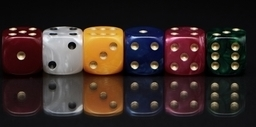The complexity of the game is entirely dependent upon the age group and math mastery of the class, making it an incredibly versatile game for students of all ages. And because the components of the game are inexpensive, it's one of the best math games to share with families.

## Math Dice and Operations

Younger students can use Math Dice to create simple addition and subtraction equations following these steps:

• Ask students to roll the target dice (one or both, depending on student mastery) to get a target number.
• Have students roll two or all three of the scoring dice and add them together to see how close they come to the target number.

If students are doing more complex equations or subtraction, they can use all three dice and create an equation by adding or subtracting numbers to get as close to the target dice number as possible.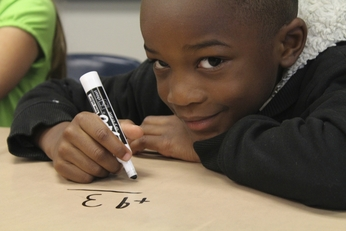### Multiplication and Division

For students who have mastered addition and subtraction and are ready to move on to multiplication and division, all five dice will be necessary. A more complex game would be played like this:

• Have students roll the two target dice and multiply the numbers to come up with a target number.
• Instruct students to roll the three scoring dice and use the numbers to create multiple equations that will get them as close to that target number as possible.
• Encourage students to look at more than one way the numbers can be used to achieve the desired results.

By creating flashcards that students can use with the dice, it becomes possible to drill them on the concept of order of operation as well.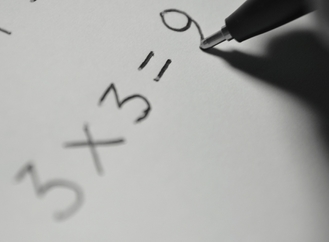Teachers can set the game up in multiple ways to benefit a variety of learning groups. With two students playing, teachers can assign one student the responsibility of calculating the target number, while the other student figures out the scoring equation. With multiple students, teachers can challenge them to come up with as many ways as possible to get as close to the target number as they can, awarding a point to the student who gets the closest.

### Higher Math

Math Dice can also be used with powers and roots for students working on complex or algebraic equations. This is accomplished by using the digits on the scoring dice to represent powers or roots, rather than as a whole digit on its own. For example, if the target number is 15, and the scoring dice numbers are 3, 6, and 2, a student could build an equation that reads, 32 + 6. Since 32 = 9, by adding 6, a student can reach the target number.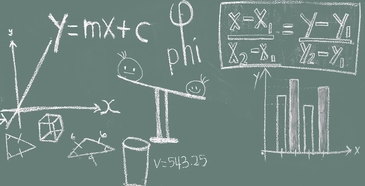Calculating square roots uses much the same principle. For example, if the target number is 6, and the scoring numbers are 2, 3, and 3, students could add the two '3s' to make 6 and use the '2' to create 62, which is 36.

Teachers can make the process more or less complicated by setting limitations on the number of players and types of mathematics (limiting students to one addition, one subtraction, one multiplication, or one division operation per equation), or by setting a timer to create a sense of urgency.

## Benefits of Math Games

As TeachThought notes in their article on the benefits of game-based learning, students retain more information when they're having fun. By introducing engaging games like Math Dice into the classroom, students will not only learn to retain more information, but also come to see learning as an entertaining adventure, as opposed to a job that must be completed.

That's a goal we can all shoot for.

teacher tips & tricks

Support